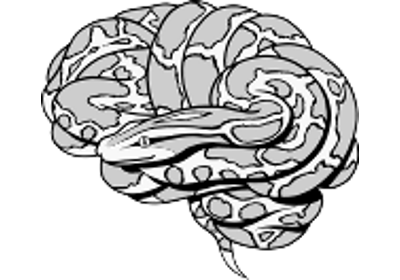Note

This page is a reference documentation. It only explains the function signature, and not how to use it. Please refer to the user guide for the big picture.

# nilearn.glm.first_level.run_glm#

nilearn.glm.first_level.run_glm(Y, X, noise_model='ar1', bins=100, n_jobs=1, verbose=0, random_state=None)[source]#

GLM fit for an fMRI data matrix.

Parameters:
Yarray of shape (n_time_points, n_voxels)

The fMRI data.

Xarray of shape (n_time_points, n_regressors)

The design matrix.

noise_model{‘ar(N)’, ‘ols’}, optional

The temporal variance model. To specify the order of an autoregressive model place the order after the characters ar, for example to specify a third order model use ar3. Default=’ar1’.

binsint, optional

Maximum number of discrete bins for the AR coef histogram. If an autoregressive model with order greater than one is specified then adaptive quantification is performed and the coefficients will be clustered via K-means with bins number of clusters. Default=100.

n_jobsint, optional

The number of CPUs to use to do the computation. -1 means ‘all CPUs’. Default=1.

verboseint, optional

The verbosity level. Default=0.

random_stateint or numpy.random.RandomState, optional

Random state seed to sklearn.cluster.KMeans for autoregressive models of order at least 2 (‘ar(N)’ with n >= 2). Default=None.

New in version 0.9.1.

Returns:
labelsarray of shape (n_voxels,),

A map of values on voxels used to identify the corresponding model.

resultsdict,

Keys correspond to the different labels values values are RegressionResults instances corresponding to the voxels.

## Examples using `nilearn.glm.first_level.run_glm`#Example of surface-based first-level analysis

Example of surface-based first-level analysisSurface-based dataset first and second level analysis of a dataset

Surface-based dataset first and second level analysis of a dataset